# NCERT Solutions for Class 9 Maths Exercise 10.2## myCBSEguide App

CBSE, NCERT, JEE Main, NEET-UG, NDA, Exam Papers, Question Bank, NCERT Solutions, Exemplars, Revision Notes, Free Videos, MCQ Tests & more.

NCERT solutions for Class 9 Maths Circles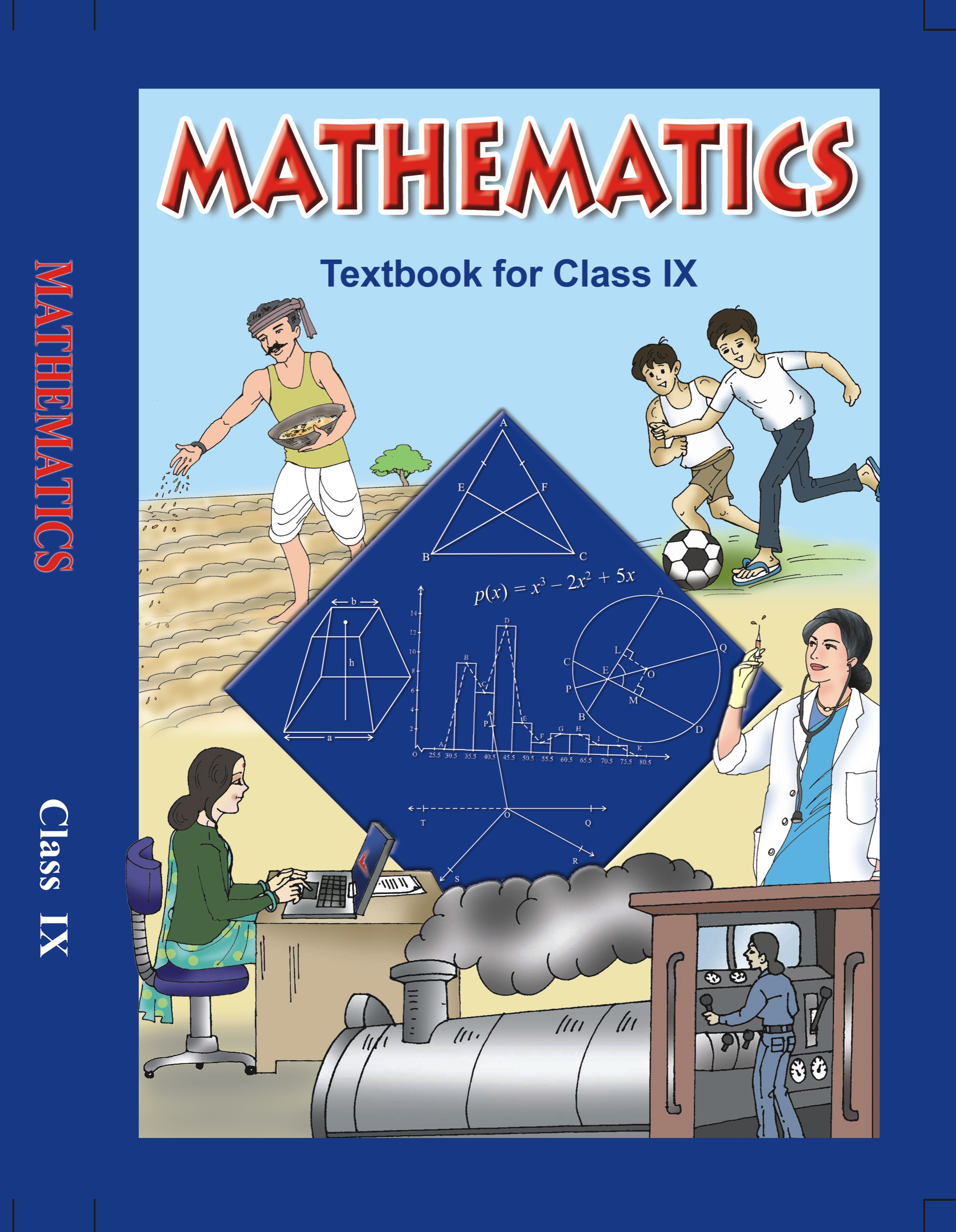## NCERT Solutions for Class 9 Mathematics Circles

###### 1. Recall that two circles are congruent if they have the same radii. Prove that equal chords of congruent circles subtend equal angles at their centres.

Ans. I Part: Two circles are said to be congruent if and only if one of them can be superposed on the other so as to cover it exactly.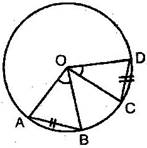Let C (O,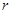) and C (O’,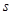) be two circles. Let us imagine that the circle C (O’,) is superposed on C (O,) so that O’ coincide with O. Then it can easily be seen that C (O’,) will cover C (O,) completely if and only if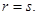Hence we can say that two circles are congruent, if and only if they have equal radii.

II Part: Given: In a circle (O,), AB and CD are two equal chords, subtend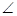AOB andCOB at the centre.

To Prove:AOB =COD

Proof: In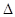AOB andCOD,

AB = CD[Given]

AO = CO[Radii of the same circle]

BO = DO [Radii of the same circle]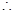AOB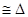COD [By SSS axiom]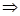AOB =COD [By CPCT]

Hence Proved.

NCERT Solutions for Class 9 Maths Exercise 10.2

###### 2. Prove that if chords of congruent circles subtend equal angles at their centres, then the chords are equal.

Ans. Given: In a circle (O,), AB and CD subtend two angles at the centre such thatAOB =COD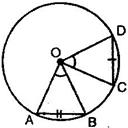To Prove: AB = CD

Proof: : InAOB andCOD,

AO = CO [Radii of the same circle]

BO = DO [Radii of the same circle]AOB =COD [Given]AOBCOD [By SAS axiom]AB = CD [By CPCT]

Hence proved.

## NCERT Solutions for Class 9 Maths Exercise 10.2

NCERT Solutions for Class 9 Maths PDF (Download) Free from myCBSEguide app and myCBSEguide website. Ncert solution class 9 Maths includes text book solutions from Mathematics Book. NCERT Solutions for CBSE Class 9 Maths have total 15 chapters. 9 Maths NCERT Solutions in PDF for free Download on our website. Ncert Maths class 9 solutions PDF and Maths ncert class 9 PDF solutions with latest modifications and as per the latest CBSE syllabus are only available in myCBSEguide.

## CBSE app for Class 9

To download NCERT Solutions for Class 9 Maths, Computer Science, Home Science,Hindi ,English, Social Science do check myCBSEguide app or website. myCBSEguide provides sample papers with solution, test papers for chapter-wise practice, NCERT solutions, NCERT Exemplar solutions, quick revision notes for ready reference, CBSE guess papers and CBSE important question papers. Sample Paper all are made available through the best app for CBSE students and myCBSEguide website.